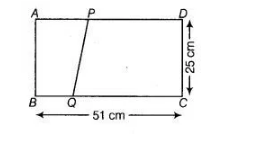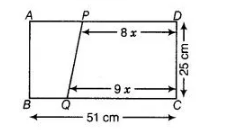# The dimensions of a rectangle ABCD are 51 cm x 25 cm.`
Question:

The dimensions of a rectangle ABCD are 51 cm x 25 cm. A trapezium PQCD with its parallel sides QC and PD in the ratio 9 : 8, is cut off from the

rectangle as shown in the figure. If the area of the trapezium PQCD is 5/6 th part of the area of the rectangle. Find the lengths QC and PD.Solution:

We have dimensions of a recstangle $A B C D$ as $51 \mathrm{~cm}$ and $25 \mathrm{~cm}$. Also, in trapezium $P Q C D$, parallel sides $Q C$ and $P D$ are in the ratio $9: 8$.

i.e.n  $Q C: P D=9: 8$

Let, length   $Q C=9 x$

and $P D=8 x$According to the question,

Area of trapezium $P Q C D=\frac{5}{6}$ area of rectangle $A B C D$

$\Rightarrow \frac{1}{2}$ (Sum of parallel sides) $\times$ Distance between parallel sides $=\frac{5}{6} \times(B C \times C D)$

$\Rightarrow \quad \frac{1}{2}(8 x+9 x) \times 25=\frac{5}{6} \times 51 \times 25$

$\Rightarrow \quad \frac{1}{2} \times 17 x \times 25=\frac{5}{6} \times 51 \times 25$

$\Rightarrow \quad x=\frac{5 \times 51 \times 25 \times 2}{25 \times 17 \times 6}$

$\Rightarrow \quad x=5$

$\therefore$ Lengths, $\quad Q C=9 \times 5=45 \mathrm{~cm}$

and $\quad P D=8 \times 5=40 \mathrm{~cm}$.